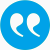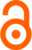Grand, Alexandra, Dittrich, Regina. 2015. Modelling assumed metric paired comparison data: Application to learning related emotions. Austrian Journal of Statistics 44 (1): S. 3-15.Show publication in ePubWU

BibTeX

Abstract

In this article we suggest a beta regression model that accounts for the degree of preference in paired comparisons measured on a bounded metric paired comparison scale. The beta distribution for bounded continuous random variables assumes values in the open unit interval (0, 1). However, in practice we will observe paired comparison responses that lie within a fixed or arbitrary fixed interval [-a, a] with known value of a. We therefore transform the observed responses into the interval (0, 1) and assume that these transformed responses are each a realization of a random variable which follows a beta distribution. We propose a simple paired comparison regression model for beta distributed variables which allows us to model the mean of the transformed response using a linear predictor and a logit link function where the linear predictor is defined by the parameters of the logit-linear Bradley Terry model. For illustration we applied the presented model to a data set obtained from a student survey of learning related emotions in mathematics.

Tags

Press 'enter' for creating the tag

Publication's profile

Status of publication Published WU Journal article Austrian Journal of Statistics English Modelling assumed metric paired comparison data: Application to learning related emotions. 44 1 2015 3 15 Y http://www.ajs.or.at/index.php/ajs/article/view/vol44-1-1 http://dx.doi.org/10.17713/ajs.v44i1.25

Associations

Projects
The analysis of rank order preference data based on Bradley-Terry type models
People
Dittrich, Regina (Former researcher)
External
Grand, Alexandra (Wirtschaftsuniversität Wien, Austria)
Organization
Institute for Statistics and Mathematics IN (Details)
Competence Center for Empirical Research Methods WE (Details)